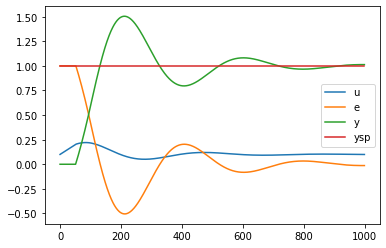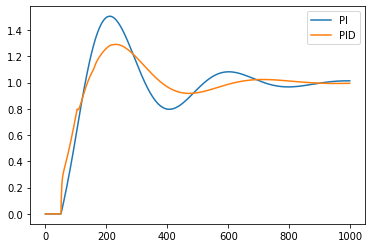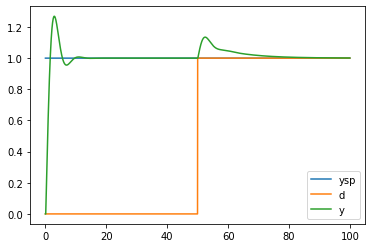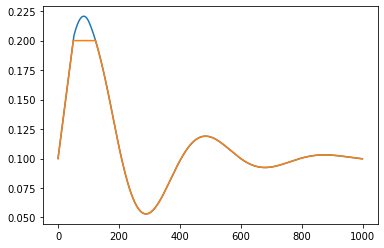# 97. Blocksim¶

tbcontrol.blocksim is a simple library for simulating the kinds of block diagrams you would encounter in a typical undergraduate control textbook. Let’s start with the most basic example of feedback control.:

import tbcontrol
tbcontrol.expectversion("0.1.1")

:

from tbcontrol import blocksim


Our first job is to define objects representing each of the blocks. A common one is the LTI block

:

Gp = blocksim.LTI('Gp', 'u', 'y', 10, [100, 1], 50)

:

Gp

:

LTI: u →[ Gp ]→ y


We’ll use a PI controller

:

Gc = blocksim.PI('Gc', 'e', 'u', 0.1, 50)

:

Gc

:

PI: e →[ Gc ]→ u


Once we have the blocks, we can create a Diagram.

Sums are specified as a dictionary with the keys being the output signal and the values being a tuple containing the input signals. The leading + is compulsory.

The inputs come next and are specified as functions of time. Blocksim.step() can be used to build a step function.

:

diagram = blocksim.Diagram([Gp, Gc],
sums={'e': ('+ysp', '-y')},
inputs={'ysp': blocksim.step()})

:

diagram

:

LTI: u →[ Gp ]→ y
PI: e →[ Gc ]→ u


Blocksim is primarily focused on being able to simulate a diagram. The next step is to create a time vector and do the simulation.

:

import numpy


The time vector also specifies the step size for integration. Since blocksim uses Euler integration internally you should choose a time step which is at least 10 times smaller than the smallest time constant of all the blocks. The timespan is of course dependent on what you are investigating.

:

ts = numpy.arange(start=0, stop=1000, step=1)

:

simulation_results = diagram.simulate(ts, progress=True)




The result of simulate() is a dictionary containing the simulation results.

:

import matplotlib.pyplot as plt

:

%matplotlib inline

:

for signal, value in simulation_results.items():
plt.plot(ts, value, label=signal)
plt.legend()

:

<matplotlib.legend.Legend at 0x1c19f872b0>## 97.1. Re-using parts of a diagram¶

Let’s compare the output of a PI and a PID controller on this system. We’ve already got the PI response, which we should store.

:

y_pi = simulation_results['y']


Let’s swap out the PI controller for a PID.

:

Gc_pid = blocksim.PID('Gc', 'e', 'u', 0.1, 50, 25)

:

diagram.blocks = [Gp, Gc_pid]

:

simulation_results = diagram.simulate(ts, progress=True)



:

plt.plot(ts, y_pi, label='PI')
plt.plot(ts, simulation_results['y'], label='PID')
plt.legend()

:

<matplotlib.legend.Legend at 0x1c1a113d68>We can see that adding the derivative action has improved control.

# 98. Disturbances¶

We can simulate a more complicated block diagram with a disturbance.:

Km = blocksim.LTI('Km', 'ysp', 'ytildesp', 1, 1)
Gc = blocksim.PI('Gp', 'e', 'p', Kc=8, tau_i=10)
Gv = blocksim.LTI('Gv', 'p', 'u', 1, 1)
Gp = blocksim.LTI('Gp', 'u', 'yu', , [10, 1])
Gd = blocksim.LTI('Gd', 'd', 'yd', , [10, 1])
Gm = blocksim.LTI('Gm', 'y', 'ym', , [1, 1])

blocks = [Km, Gc, Gv, Gp, Gd, Gm]

:

sums = {'e': ('+ytildesp', '-ym'),
'y': ('+yd', '+yu')}

inputs = {'ysp': blocksim.step(),
'd': blocksim.step(starttime=50)}

:

diagram = blocksim.Diagram(blocks, sums, inputs)

:

ts = numpy.arange(start=0, stop=100, step=0.05)

:

results = diagram.simulate(ts, progress=True)



:

for name in ('ysp', 'd', 'y'):
plt.plot(ts, results[name], label=name)
plt.legend()

:

<matplotlib.legend.Legend at 0x1c19feccf8># 99. Algebraic equations¶

Sometimes it is useful to be able to handle non-linear calculations in block diagrams. This deviates from the strict interpretation of block diagrams but can be useful for instance in calculating the response of a controller with output limits.

:

import numpy

:

def limit(t, u):
return numpy.clip(u, 0, 0.2)

:

Gp = blocksim.LTI('Gp', 'ulimited', 'y', 10, [100, 1], 50)
Gc = blocksim.PI('Gc', 'e', 'u', 0.1, 50)

:

limiter = blocksim.AlgebraicEquation('Limiter', 'u', 'ulimited', limit)

:

diagram = blocksim.Diagram([Gp, Gc, limiter],
sums={'e': ('+ysp', '-y')},
inputs={'ysp': blocksim.step()})

:

ts = numpy.arange(start=0, stop=1000, step=1)

:

simulation_results = diagram.simulate(ts)

:

diagram

:

LTI: ulimited →[ Gp ]→ y
PI: e →[ Gc ]→ u
AlgebraicEquation: u →[ Limiter ]→ ulimited

:

plt.plot(ts, simulation_results['u'])
plt.plot(ts, simulation_results['ulimited'])

:

[<matplotlib.lines.Line2D at 0x1c1a16ad68>]We can see the effect of the limiter clearly in the above figure

[ ]: